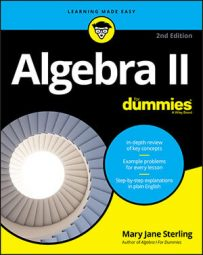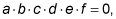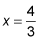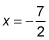##### Algebra II For DummiesLike quadratic binomials, a quadratic trinomial can have as many as two solutions — or it may have one solution or no solution at all. If you can factor the trinomial and use the multiplication property of zero to solve for the roots, you're home free.

The multiplication property of zero states that if the product ofthen at least one of the factors has to represent the number 0.

Here's an example: How many solutions can you find for the trinomial x2 – 2x – 15 = 0?

You start by factoring the left side of the equation into (x – 5)(x + 3) = 0 and then setting each factor equal to zero. When x – 5 = 0, x = 5, and when x + 3 = 0, x = –3. So, x2 – 2x – 15 = 0 has two solutions.

It may not be immediately apparent how you should factor a seemingly complicated trinomial like 24x2 + 52x – 112 = 0. Before you bail out and go to the quadratic formula, consider factoring 4 out of each term to simplify the picture a bit; you get

4(6x2 +13x – 28) = 0

The quadratic in the parentheses factors into the product of two binomials (with some educated guessing or multiplying 6x2 times –28 and finding which factors of 168x2 have a sum of 13x), giving you

4(3x – 4)(2x + 7) = 0

Setting 3x – 4 equal to 0, you getand setting 2x + 7 equal to 0, you getHow about the factor of 4? If you set 4 equal to 0, you get a false statement, which is fine; you already have the two numbers that make the equation a true statement.